### Home > CALC > Chapter 11 > Lesson 11.3.1 > Problem11-101

11-101.
1. Multiple Choice: A particle moves on a plane curve so that at any time t > 0 its coordinates are given by x = (2t − 1)4, y = t2 + 1. The acceleration of the particle at t = 1 is: Homework Help ✎

1.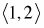2.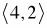3.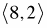4.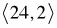5.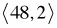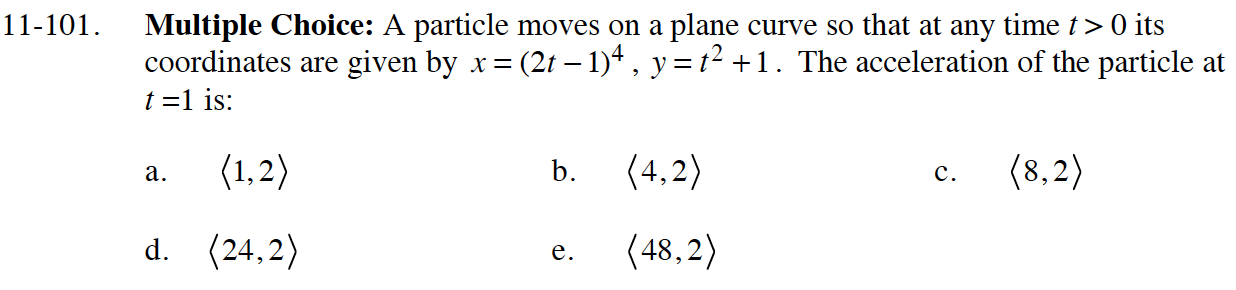$\vec{a}(1)=\langle{x^{\prime\prime}(t),y^{\prime\prime}(t)}\rangle\Big|_{t=1}$